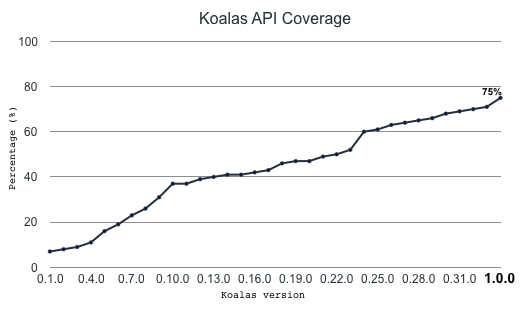# Version 1.0.0¶

## Better pandas API coverage¶

We implemented many APIs and features equivalent with pandas such as plotting, grouping, windowing, I/O, and transformation, and now Koalas reaches the pandas API coverage close to 80% in Koalas 1.0.0.## Apache Spark 3.0¶

Apache Spark 3.0 is now supported in Koalas 1.0 (#1586, #1558). Koalas does not require any change to use Spark 3.0. Apache Spark has more than 3400 fixes landed in Spark 3.0 and Koalas shares the most of fixes in many other components.

It also brings the performance improvement in Koalas APIs that execute Python native functions internally via pandas UDFs, for example, DataFrame.apply and DataFrame.apply_batch (#1508).

## Python 3.8¶

With Apache Spark 3.0, Koalas supports the latest Python 3.8 which has many significant improvements (#1587), see also Python 3.8.0 release notes.

## Spark accessor¶

spark accessor was introduced from Koalas 1.0.0 in order for the Koalas users to leverage the existing PySpark APIs more easily (#1530). For example, you can apply the PySpark functions as below:

import databricks.koalas as ks
import pyspark.sql.functions as F

kss = ks.Series([1, 2, 3, 4])
kss.spark.apply(lambda s: F.collect_list(s))


## Better type hint support¶

In the early versions, it was required to use Koalas instances as the return type hints for the functions that return a pandas instances, which looks slightly awkward.

def pandas_div(pdf) -> koalas.DataFrame[float, float]:
# pdf is a pandas DataFrame,
return pdf[['B', 'C']] / pdf[['B', 'C']]

df = ks.DataFrame({'A': ['a', 'a', 'b'], 'B': [1, 2, 3], 'C': [4, 6, 5]})
df.groupby('A').apply(pandas_div)


In Koalas 1.0.0 with Python 3.7+, you can also use pandas instances in the return type as below:

def pandas_div(pdf) -> pandas.DataFrame[float, float]:
return pdf[['B', 'C']] / pdf[['B', 'C']]


In addition, the new type hinting is experimentally introduced in order to allow users to specify column names in the type hints as below (#1577):

def pandas_div(pdf) -> pandas.DataFrame['B': float, 'C': float]:
return pdf[['B', 'C']] / pdf[['B', 'C']]


## Wider support of in-place update¶

Previously in-place updates happen only within each DataFrame or Series, but now the behavior follows pandas in-place updates and the update of one side also updates the other side (#1592).

For example, the following updates kdf as well.

kdf = ks.DataFrame({"x": [np.nan, 2, 3, 4, np.nan, 6]})
kser = kdf.x
kser.fillna(0, inplace=True)

kdf = ks.DataFrame({"x": [np.nan, 2, 3, 4, np.nan, 6]})
kser = kdf.x
kser.loc = 30

kdf = ks.DataFrame({"x": [np.nan, 2, 3, 4, np.nan, 6]})
kser = kdf.x
kdf.loc[2, 'x'] = 30


If the DataFrame and Series are connected, the in-place updates update each other.

## Less restriction on compute.ops_on_diff_frames¶

In Koalas 1.0.0, the restriction of compute.ops_on_diff_frames became much more loosened (#1522, #1554). For example, the operations such as below can be performed without enabling compute.ops_on_diff_frames, which can be expensive due to the shuffle under the hood.

df + df + df
df['foo'] = df['bar']['baz']
df[['x', 'y']] = df[['x', 'y']].fillna(0)


## Other new features and improvements¶

DataFrame:

• __bool__ (#1526)

• explode (#1507)

• spark.apply (#1536)

• spark.schema (#1530)

• spark.print_schema (#1530)

• spark.frame (#1530)

• spark.cache (#1530)

• spark.persist (#1530)

• spark.hint (#1530)

• spark.to_table (#1530)

• spark.to_spark_io (#1530)

• spark.explain (#1530)

• spark.apply (#1530)

• mad (#1538)

• __abs__ (#1561)

Series:

• item (#1502, #1518)

• divmod (#1397)

• rdivmod (#1397)

• unstack (#1501)

• mad (#1503)

• __bool__ (#1526)

• to_markdown (#1510)

• spark.apply (#1536)

• spark.data_type (#1530)

• spark.nullable (#1530)

• spark.column (#1530)

• spark.transform (#1530)

• filter (#1511)

• __abs__ (#1561)

• bfill (#1580)

• ffill (#1580)

Index:

• __bool__ (#1526)

• spark.data_type (#1530)

• spark.column (#1530)

• spark.transform (#1530)

• get_level_values (#1517)

• delete (#1165)

• __abs__ (#1561)

• holds_integer (#1547)

MultiIndex:

• __bool__ (#1526)

• spark.data_type (#1530)

• spark.column (#1530)

• spark.transform (#1530)

• get_level_values (#1517)

• delete (#1165

• __abs__ (#1561)

• holds_integer (#1547)

Along with the following improvements:

• Fix Series.clip not to create a new DataFrame. (#1525)

• Fix combine_first to support tupled names. (#1534)

• Add Spark accessors to usage logging. (#1540)

• Implements multi-index support in Dataframe.filter (#1512)

• Fix Series.fillna to avoid Spark jobs. (#1550)

• Support DataFrame.spark.explain(extended: str) case. (#1563)

• Support Series as repeats in Series.repeat. (#1573)

• Fix fillna to handle NaN properly. (#1572)

• Fix DataFrame.replace to avoid creating a new Spark DataFrame. (#1575)

• Cache an internal pandas object to avoid run twice in Jupyter. (#1564)

• Fix Series.div when div/floordiv np.inf by zero (#1463)

• Fix Series.unstack to support non-numeric type and keep the names (#1527)

• Fix hasnans to follow the modified column. (#1532)

• Fix explode to use internal methods. (#1538)

• Fix RollingGroupby and ExpandingGroupby to handle agg_columns. (#1546)

• Fix reindex not to update internal. (#1582)

## Backward Compatibility¶

• Remove the deprecated pandas_wraps (#1529)

• Remove compute function. (#1531)